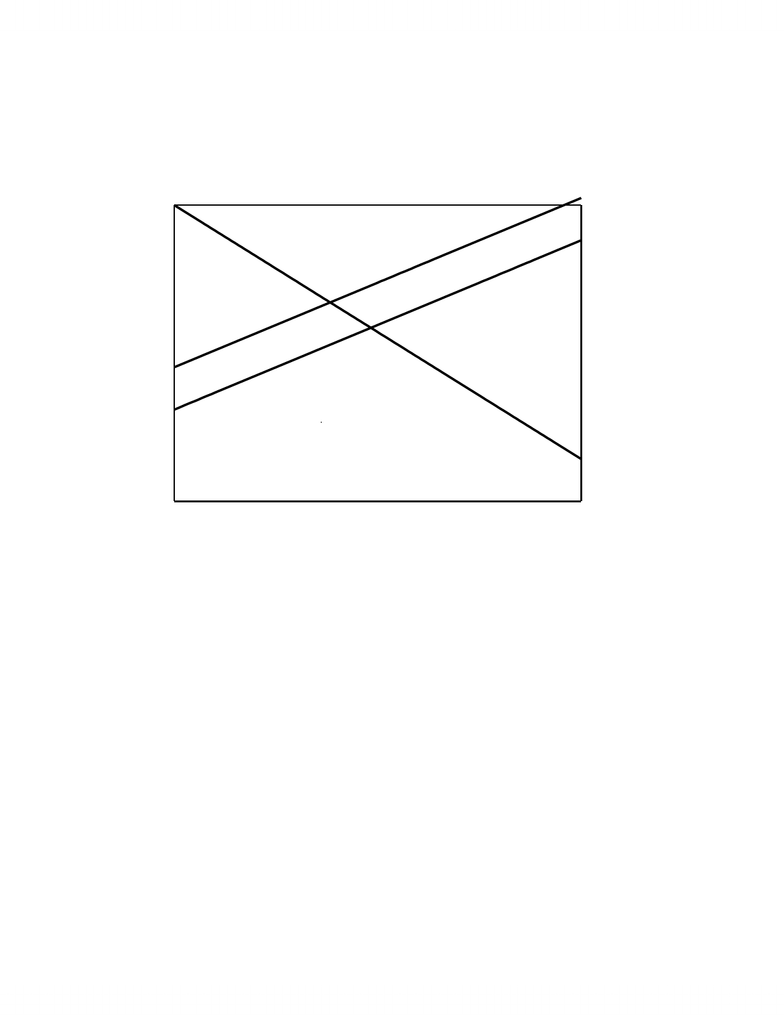# BU472 Lecture Notes - Remittance, Economic Equilibrium

36 views7 pagesPrinciples of Microeconomics: Sales Taxes and Subsidies
SALES TAXES
Our understanding of elasticity will help us analyze the impact of sales taxes or subsidies on
equilibrium price and quantity.
1. Per Unit Tax
The simplest sales tax is a fixed tax per unit sold, regardless of the price or quantity of the
units sold. Whether sellers or buyers collect the tax for remission to the government is irrelevant
to the impact of the tax on buyers and sellers but we will assume that sellers collect the tax since
this is the more common occurrence due to the greater ease of remission. Since the sellers
impose the tax on each unit sold, the sellers add the amount of the tax to the price of each unit
sold. This means that the supply function shifts up by the amount of the tax.
If the equation for supply was Ps = S(Q), the PsTax = T + S(Q) where T = tax/unit.
e.g. Suppose P = 84 – 0.03Q and P = 26 + 0.02Q describe the market for opera tickets per day.
Initial Equilibrium => 84 – 0.03Q = 26 + 0.02Q
=> Qo = 1160 and Po = \$49.20
What is the effect (short-run) of a tax of \$12/unit?
=> Supply: P = 12 + 26 + 0.02Q
After Tax Equilibrium => 84 – 0.03Q = 38 + 0.02Q
=> Q1 = 920 and P1 = \$56.40
Total Tax Revenue = Tax/unit * Q1 = \$3 * 1100 = \$3300
NOTE: The equilibrium price after the tax increased by \$7.20 (\$56.40 - \$49.20) not \$12. A
graph of these equilibria helps us understand why.
Graph the after-tax equilibrium by shifting up the supply curve by the amount of the tax.
Indicate the original price plus the tax (P0+T) on the vertical axis and shift supply up at Qo by this
- 1 -
Unlock document

This preview shows pages 1-2 of the document.
Unlock all 7 pages and 3 million more documents.Principles of Microeconomics: Sales Taxes and Subsidies
amount. Most individuals shift supply up to intersect D at P0+T but this is incorrect since Supply
clearly shifts by more than the amount of the tax at that point.
P = 84 - 0.02Q, P = 26 + 0.03Q, Tax = \$12/unit
0
200
400
600
800
1000
1200
1400
1600
1800
2000
2200
2400
0
20
40
60
80
Price (\$)
So
D
S
1
Po
Qo
P
1
Q1
Po+t
Quantity
Sellers add the tax to the price that they charge customers but customers do continue to buy
Qo at the higher price due to the Law of Demand. The higher price results in a fall in quantity
demanded leading to a surplus at P0+T, which causes a fall in price to a new equilibrium P1 that is
usually less than P0+T though greater than Po. The increase in price and decrease in quantity as a
result of the tax is a function of the relative elasticities of Demand and Supply. We will see later
that a comparison of the relative slopes of Demand and Supply suffices to determine the change
in price for linear Demand.
- 2 -
Unlock document

This preview shows pages 1-2 of the document.
Unlock all 7 pages and 3 million more documents.

# Get access

\$10 USD/m
Billed \$120 USD annually
Homework Help
Class Notes
Textbook Notes